@lutingting 2016-11-07T00:52:04.000000Z 字数 5512 阅读 11415

# Hough变换原始形式-直线检测

数字图像处理 图像特征提取

# 1.直角坐标系与极坐标系中的直线表示

## 1.1 直角坐标系与极坐标系 中的点的表示及它们之间相互关系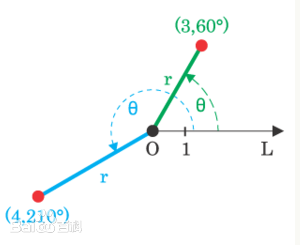• 1.在直角坐标系下，点P的坐标表示为$(x,y)$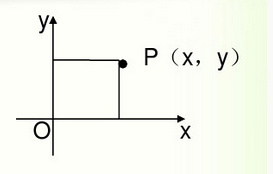• 2.在极坐标系下，点的坐标表示为$(\rho,theta)$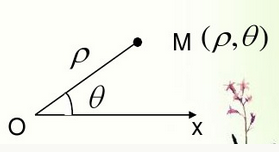• 3.某一点由极坐标转换为直角坐标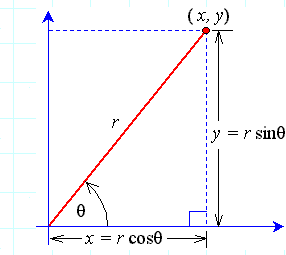注：图中的r与上面的$\rho$是同样的描述

• 4.某一点直角坐标转换为极坐标

• $\theta$的具体取值还需要根据点P具体位于哪个象限进行调整，根据点的直角坐标可以判断其位于哪个象限，具体判断方法如下图如示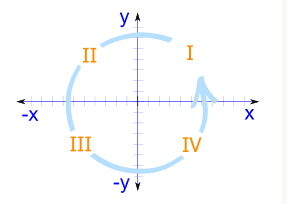• 根据点具体落到四个象限中的哪一个，在arctan结果 的基础上进行修正，arctan结果位于(-π/2,π/2)区间，具体方法如下：
• 点位于第一象限，则该点的极角为arctan
• 点位于第二象限，则该点的极角为arctan+π
• 点位于第三象限，则该点的极角为arctan+π
• 点位于第四象限，则该点的极角为arctan+2## 1.2 直角坐标系与极坐标系中的直线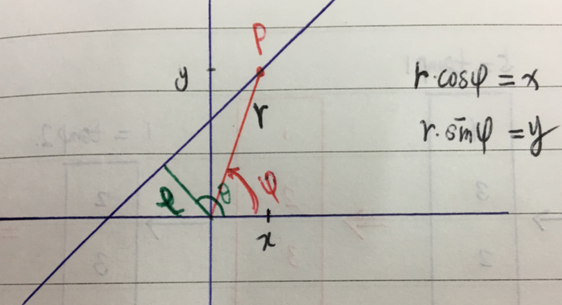• 首先，利用直线距离原点的距离的计算公式，可得下式
• 然后，对该式利用三角函数展开，可以得到
• 又根据点P的直角坐标系(x,y)与极坐标 $( \varphi,r )$之间 的关系$r * cos(\varphi) = x$$r * sin(\varphi) = y$，可以得到

# 2.利用Hough变换检测直线

## 2.1 线到点的Hough变换

### 2.1.1 通俗解释

• 给定一幅图像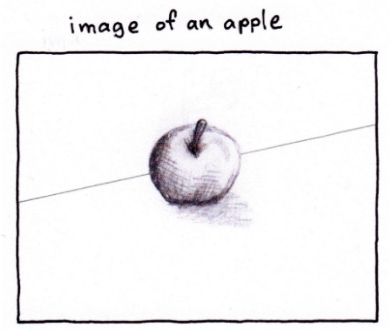• 检测边缘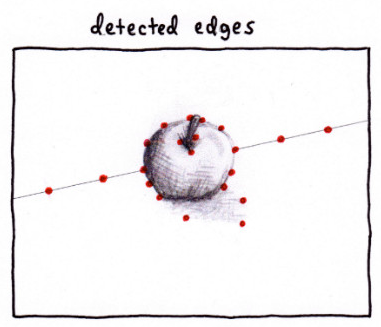• 对于任何一个边缘点，找到所有可能经过该点的直线，这些直线每一条都对应着参数空间中的一个点，而无穷多条之间对应于极坐标系下的点将形成一条极坐标系下的曲线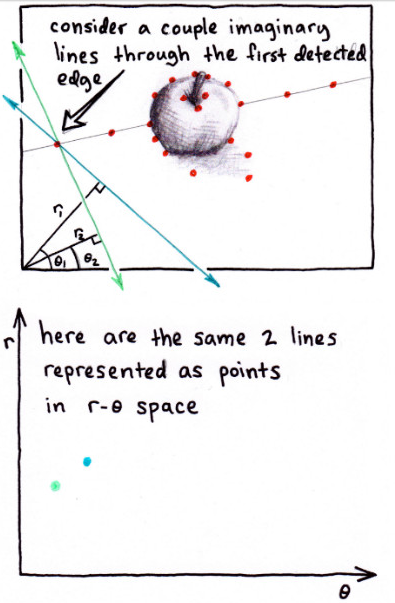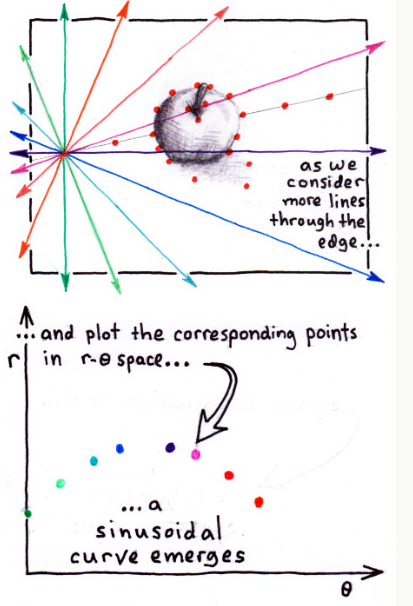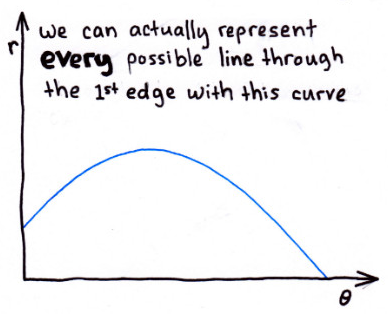• 重复上面过程，每个边缘点都对应于极坐标系下的一条曲线，那么，极坐标系下 （参数空间）所有这些曲线的交点一定是原图像中所有边缘点共同存在的直线！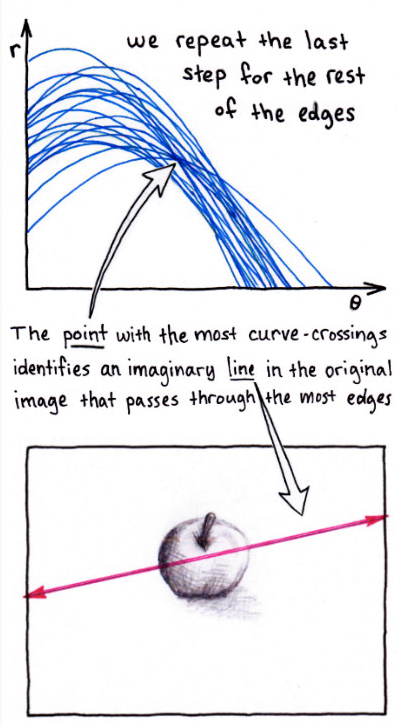## 2.2 Hough变换检测直线的实现

### 2.2.1 赵小川教程中的例子

• 假设一副图像大小为$M\times M$，根据该图像尺寸，设定hough变换的参数空间取值范围
• 原点距离直线的距离：$\rho \in [-\sqrt{2}N,\sqrt{2}N]$
• 原点到直线的垂线与x轴正方向的夹角：$\theta \in {[0,\pi] }$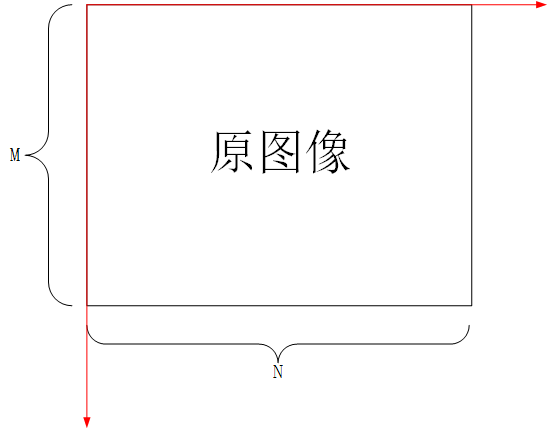• 按照参数的取值范围，将参数分为$m \times n$个网格，即将$\theta \in {[0,\pi] }$分为m份，将$\rho \in [-\sqrt{2}N,\sqrt{2}N]$分为n份，然后，设定一个$m \times n$的累加单元，用来存储图像中某一条直线出现的次数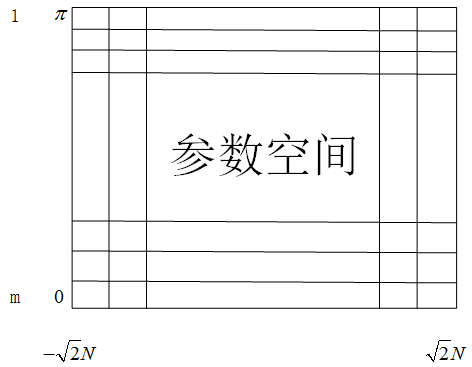• 接下来，对图像中原图像中每一个像素点(x,y)，分别进行如下操作：

• 在参数$\theta$对应的每一取值，分别按照公式$\rho = xcos\theta +ysin\theta$计算相应的参数$\rho$的取值
• 然后，在相应的参数累加单元中加1
• 按照上面操作，得到了一个累加单元，统计每个累加单元的取值，大于某个事先设定好的阈值，就认为该组参数便是图像空间内的直线的参数

### 2.2.2 matlab自带hough变换相关函数

matlab自带了Hough变换的相关函数：

• hough：实现霍夫变换，得到霍夫变换矩阵，用法如下
• [H, theta, rho] = hough(BW)
• 输入BW为二值边缘图像
• 输出H为参数空间参数的累加矩阵；
• 输出theta为数值向量，存储划分参数空间的参数$\theta$的所有取值；
• 输出rho为数值向量，存储划分参数空间的参数$\rho$的所有取值
• [H, theta, rho] = hough(BW, ParameterName,ParameterValue)
• 这里增加了一组用来指定参数$\theta$$\rho$的区间范围(及间隔)
• 'RhoResolution'：指定参数$\theta$的区间的间隔
• 'ThetaResolution'：指定参数$\rho$的区间的间隔
• 'Rho'：指定参数$\rho$的区间的范围(及间隔)，例如：1:0.5:100（注：默认值为(0,norm(size(BW)))，即最大值为图像对角线长度，间隔为1）
• 'Theta'：指定参数$\theta$的区间的范围(及间隔)，例如：0:0.5:50，（注：默认值为[-90°，90°)，间隔为1，单位为度）
• houghpeaks：在霍夫变换矩阵里找极值点

• peaks = houghpeaks(H, numpeaks)：找到hough变换矩阵中最大的numpeaks个值
• peaks = houghpeaks(..., param1, val1,param2, val2)：对最大值提取进行一些设置
• 'Threshold'：大于该阈值的点才会考虑是否为峰值，默认值为$0.5*max(H(:))$
• 'NHoodSize'：非极大值抑制窗的大小，默认为$size(H)/50$
• houghlines：从霍夫变换矩阵中提取线段

• lines = houghlines(BW, theta, rho,peaks)
• BW为原始图像边缘
• theta为hough返回的参数$\theta$的区间间隔
• rho为hough返回的参数$\rho$的区间间隔
• peaks为houghpeaks提取出来的hough变换的极值点
• lines = houghlines(..., param1, val1,param2, val2)
• 输入值：'FillGap'：指定了与相同的霍夫变换相关的两条线段的距离。当两条线段之间的距离小于指定的值时，函数houghlines把线段合并为一条线段(默认的距离是20个像素)
• 输入值：'MinLength'：指定合并的线是保留还是丢弃。如果合并的线比val2指定的值短，就丢弃(默认值是40)
• 输出值：lines结构元胞，分别存放合并后的所有直线的相关信息
• point1：两元素向量[r1, c1]，指定了线段起点的行列坐标。
• point2：两元素向量[r2, c2]，指定了线段终点的行列坐标。
• theta：与线相关的霍夫变换的以度计量的角度。
• rho：与线相关的霍夫变换的ρ轴位置。

1. 读取图像并得到边缘图像

% 读取matlab自带的图像gantrycrane，并提取边缘RGB = imread('gantrycrane.png');I  = rgb2gray(RGB);BW = edge(I,'canny'); %提取边缘subplot(1,2,1);imshow(RGB);title('原始图像'); subplot(1,2,2);imshow(BW);title('边缘图像');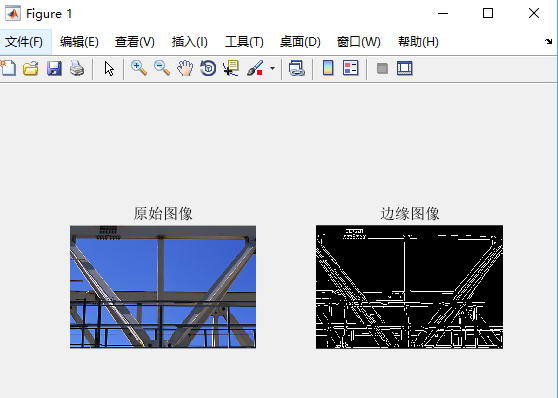2. 利用Hough函数对输入图像边缘进行hough变换

% 对原始图像边缘进行hough变换，指定参数的分别率（取样间隔），区间利用默认值[H,T,R] = hough(BW,'RhoResolution',0.5,'ThetaResolution',0.5);
• H为hough transform矩阵，每个元素分别存放的相应参数对应的累加
• T为存放theta的采样值的向量
• R为存放rho采样值的向量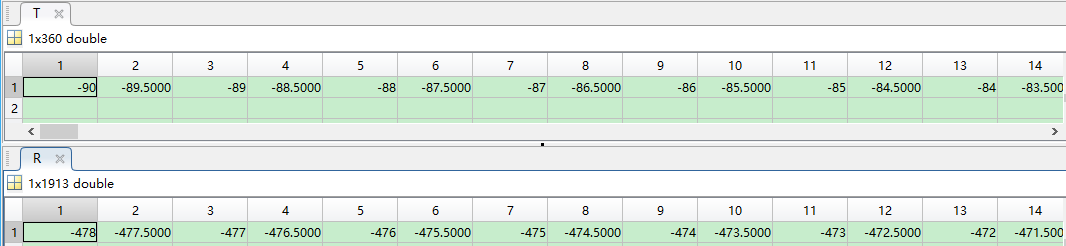3.显示hough变换的结果，即显示矩阵H（即bins）

% 显示hough变换的结果figure;imshow(imadjust(mat2gray(H)),'XData',T,'YData',R,...   'InitialMagnification','fit');title('Limited Theta Range Hough Transform of Gantrycrane Image');xlabel('\theta'), ylabel('\rho');axis on, axis normal;colormap(hot)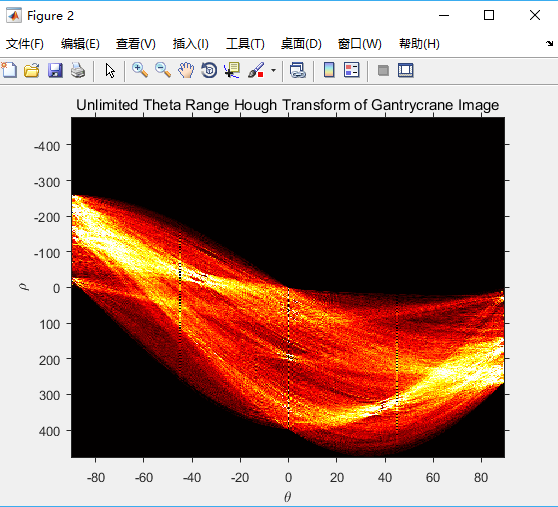[H,T,R] = hough(BW, 'Theta', 44:0.5:46);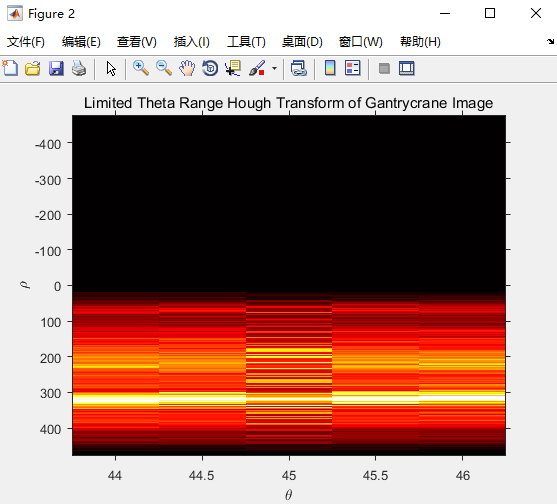4.显示hough变换结果中的极值点

% 显示霍夫变换矩阵中的极值点P = houghpeaks(H,10,'threshold',ceil(0.3*max(H(:)))); % 从霍夫变换矩阵H中提取50个极值点x = T(P(:,2));%极值点的theta值，即P的第二列存放的是极值点的theta值y = R(P(:,1));%极值点的rho值，即P的第二列存放的是极值点的rho值hold on;plot(x,y,'s','color','black');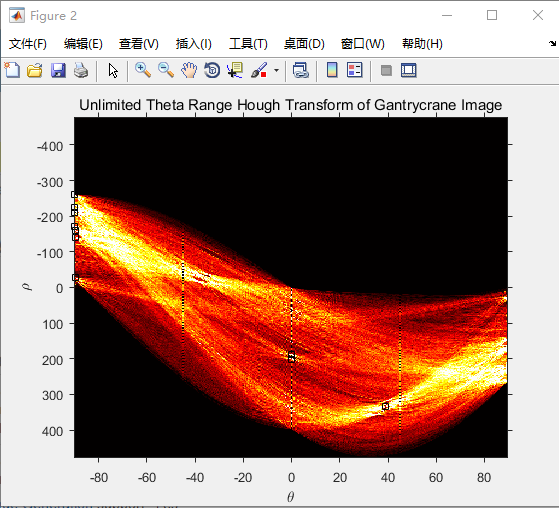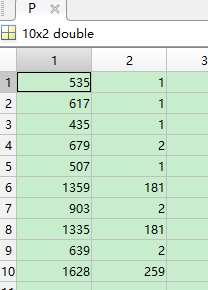5.将提取得到的极值点变换回原图像，得到提取的直线

% 找原图中的直线lines = houghlines(BW,T,R,P,'FillGap',18,'MinLength',80);

• 'FillGap'：当两条直线之间距离小于该阈值时，两条直线被合并为一条直线
• 'MinLength'：保留的直线的最短长度（即小于该阈值的直线将被删除）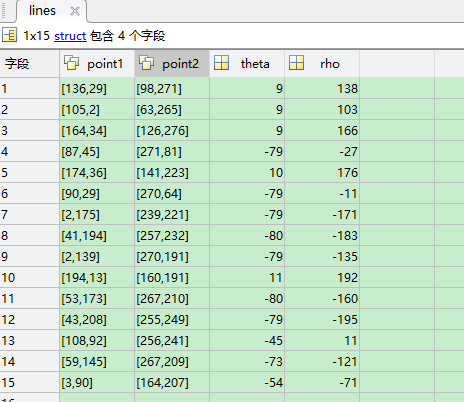6.在原图像中绘制得到的直线

% 绘制提取得到的直线figure, imshow(I), hold onmax_len = 0;for k = 1:length(lines)    % 绘制第k条直线    xy = [lines(k).point1; lines(k).point2];    plot(xy(:,1),xy(:,2),'LineWidth',2,'Color','green');    % 绘制第k条直线的起点（黄色）、终点（红色）    plot(xy(1,1),xy(1,2),'x','LineWidth',2,'Color','yellow');    plot(xy(2,1),xy(2,2),'x','LineWidth',2,'Color','red');    % 计算第k条直线的长度，保留最长直线的端点    len = norm(lines(k).point1 - lines(k).point2);    if ( len > max_len)        max_len = len;        xy_long = xy;    endendtitle('提取到的直线');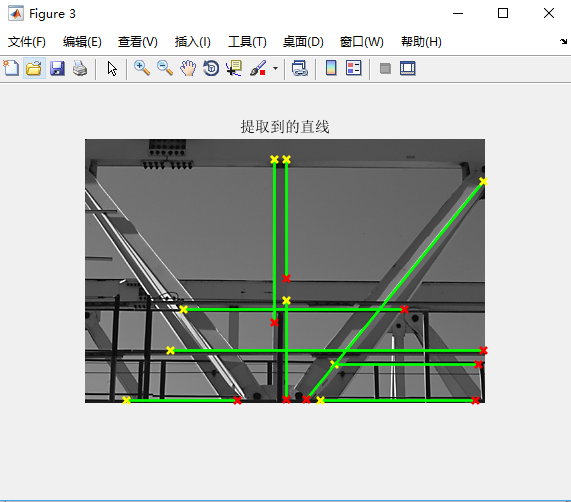% 以红色线高亮显示最长的线plot(xy_long(:,1),xy_long(:,2),'LineWidth',2,'Color','red');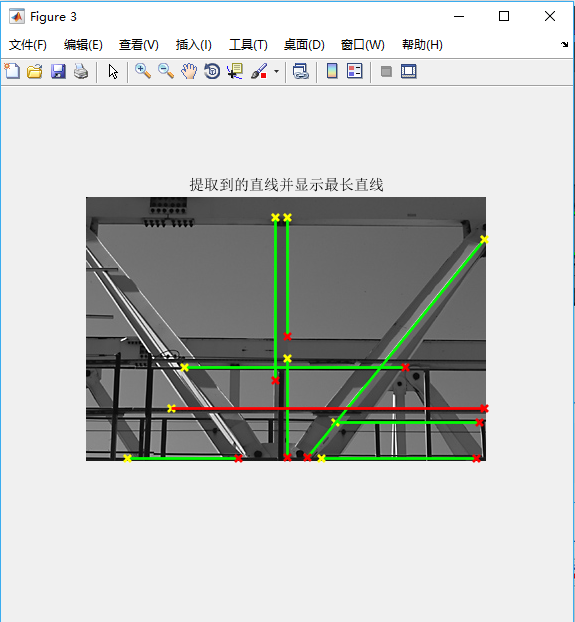# 3.Hough变换的优缺点

• 抗噪能力强
• 对边缘间断不敏感

• 运算量大：对图像中每一个像素点，都需要计算所有的theta对一个的rho
• 占用内存多

Hough变换利用的是一种投票思想

Reference

1. stackoverflow=explain hough transformation．http://stackoverflow.com/questions/4709725/explain-hough-transformation
2. Polar and Cartesian Coordinates． https://www.mathsisfun.com/polar-cartesian-coordinates.html